# WELCOME TO FUNMATHS

Funmaths.com is all about high school math.

We believe learning maths can be fun! And at high school math level too! Funmaths.com provides resources for high school teachers - math worksheets, lesson plans, fun ideas, games and puzzles covering all the advanced topics like algebra, trigonometry, calculus, statistics, geometry and more.

You will find printable math worksheets, tutorials and math review , cool math games, math puzzles and all sorts of fun math projects for high school math students.## FOR TEACHERS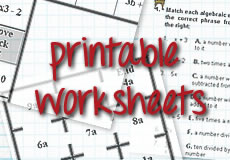### PRINTABLE WORKSHEETS

Looking for fun ideas or lesson plans for your math classroom? Print out math worksheets for classroom use. For something fun, check out the math puzzles.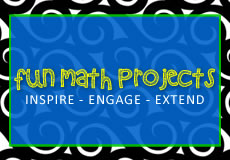### FUN MATH PROJECTS

We've got a number of great ideas for math in real life, math and art, or math for special occassions like Valentine's Day. Check on Fun Math Projects.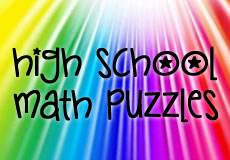### MATH GAMES & PUZZLES

Use math games & puzzles to entertain your math students. Develop the logic and math side of the brain. Create for classroom starters.

## FUN MATH DESIGNS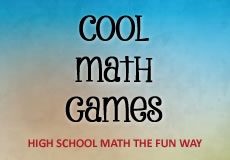### COOL MATH GAMES

Learn a new concept, develop your logic or spatial skills. A number of cool math games to turn on the math side of your brain.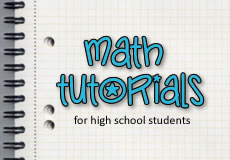### MATH TUTORIALS

This is a free resource for high school math students and for parents who would like to help your child learn math. High school math review, tutorials & problems.